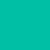# Arrow Functions in ES6 (ECMAScript 2015)

Published on 2018-01-20.

This post is for people learning JavaScript and will cover the gist of what you should know about arrow functions introduced in ES6 (ECMAScript 2015).

## Syntax of an Arrow Function

Arrow functions look like normal functions but with shorter syntax. Suppose we have the following function below:

``````function sumOf(x, y) {
return x + y;
}
console.log(sumOf(1, 2)); // 3
``````

Rewite the function using an arrow function instead:

``````var sumOf = (x, y) => x + y;
console.log(sumOf(1, 2)); // 3
``````

What follows after the arrow is what gets returned. Now that's a lot easier to read. But what if you want to do something more complicated inside your function?

``````/*
"...args" will assign args to an array
of all the arguments passed into sumOf
*/
var sumOf = (...args) => {
let sum = 0;
for (let value of args) {
sum += value;
}
return sum;
};
console.log(sumOf(1, 2, 3)); // 6
``````

To do something more than just `x + y` you can use the {}s and explicitly return what you want.

And your arrow functions don't need to have parameters; you can just have an empty set of ()s:

``````var f1 = () => "f1";
console.log(f1()); // f1
``````

## Utility of an Arrow Function

We've talked about the syntatical differences between arrow and normal functions, but what about the utility? Beneath the syntax, what sets an arrow function apart from a normal function?

There's a few differences but we're just going to go over one of them (arguably the most important one): `this`. Normal functions will define their own `this` but arrow functions do not define their own `this`.

Let's take a look at the following example:

``````var obj = {
outer: function() {
console.log(`Is "this" referring to obj? \${this === obj}`);

function inner() {
return `Is "this" referring to obj? \${this === obj}`;
}
console.log(inner());
}
};

obj.outer();

/*
Is "this" referring to obj? true
Is "this" referring to obj? false
*/
``````

Notice how the inner function has a different `this` than the outer `this`? We can stop that from happening by making the inner function an arrow function. Here's how it would look if the inner function was an arrow function:

``````var obj = {
outer: function() {
console.log(`Is "this" referring to obj? \${this === obj}`);

var inner = () => `Is "this" referring to obj? \${this === obj}`;
console.log(inner());
}
};

obj.outer();

/*
Is "this" referring to obj? true
Is "this" referring to obj? true
*/
``````

Now the `this` in both functions will refer to the object `obj`.

## Conclusion

When should you use arrow functions? Only if you stand to benefit from them in either readability (shorter syntax) or utility (functional programming, `this` in object oriented programming). Otherwise, keep rolling with normal functions.

Writing this blog post helped me understand arrow functions better. I hope this post helps others understand arrow functions too.